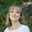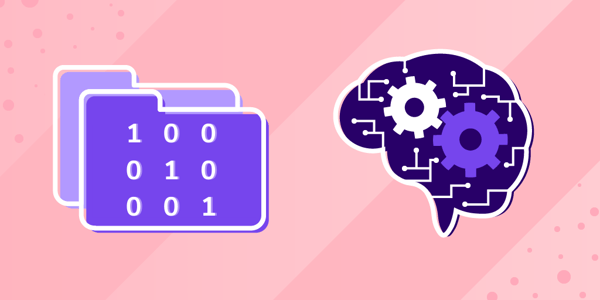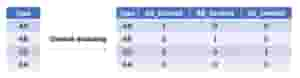# Data Science in 5 Minutes: What is One Hot Encoding?

Feb 11, 2021 - 7 min readAmanda FawcettIf you’re in the field of data science, you’ve probably heard the term “one hot encoding”. Even the Sklearn documentation tells you to “encode categorical integer features using a one-hot scheme”. But, what is one hot encoding, and why do we use it?

Most machine learning tutorials and tools require you to prepare data before it can be fit to a particular ML model. One hot encoding is a process of converting categorical data variables so they can be provided to machine learning algorithms to improve predictions. One hot encoding is a crucial part of feature engineering for machine learning.

In this guide, we will introduce you to one hot encoding and show you when to use it in your ML models. We’ll provide some real-world examples with Sklearn and Pandas.

This tutorial at a glance:

## What is one hot encoding?

Categorical data refers to variables that are made up of label values, for example, a “color” variable could have the values “red“, “blue, and “green”. Think of values like different categories that sometimes have a natural ordering to them.

Some machine learning algorithms can work directly with categorical data depending on implementation, such as a decision tree, but most require any inputs or outputs variables to be a number, or numeric in value. This means that any categorical data must be mapped to integers.

One hot encoding is one method of converting data to prepare it for an algorithm and get a better prediction. With one-hot, we convert each categorical value into a new categorical column and assign a binary value of 1 or 0 to those columns. Each integer value is represented as a binary vector. All the values are zero, and the index is marked with a 1.

Take a look at this chart for a better understanding:Let’s apply this to an example. Say we have the values red and blue. With one-hot, we would assign red with a numeric value of 0 and blue with a numeric value of 1.

It’s crucial to be consistent when we use these values. This makes it possible to invert our encoding at a later point to get our original categorical back.

Once we assign numeric values, we create a binary vector that represents our numerical values. In this case, our vector will have 2 as its length since we have 2 values. Thus, the red value can be represented with the binary vector [1,0], and the blue value will be represented as [0,1].

### Why use one hot encoding?

One hot encoding is useful for data that has no relationship to each other. Machine learning algorithms treat the order of numbers as an attribute of significance. In other words, they will read a higher number as better or more important than a lower number.

While this is helpful for some ordinal situations, some input data does not have any ranking for category values, and this can lead to issues with predictions and poor performance. That’s when one hot encoding saves the day.

One hot encoding makes our training data more useful and expressive, and it can be rescaled easily. By using numeric values, we more easily determine a probability for our values. In particular, one hot encoding is used for our output values, since it provides more nuanced predictions than single labels.

## How to convert categorical data to numerical data

Manually converting our data to numerical values includes two basic steps:

• Integer encoding
• One hot encoding

For the first step, we need to assign each category value with an integer, or numeric, value. If we had the values red, yellow, and blue, we could assign them 1, 2, and 3 respectively.

When dealing with categorical variables that have no order or relationship, we need to take this one step further. Step two involves applying one-hot encoding to the integers we just assigned. To do this, we remove the integer encoded variable and add a binary variable for each unique variable.

Above, we had three categories, or colors, so we use three binary variables. We place the value 1 as the binary variable for each color and the value 0 for the other two colors.

red,	yellow,	 blue
1,		0,		0
0,		1,		0
0,		0,		1


Note: In many other fields, binary variables are referred to as dummy variables.

### Keep the learning going.

Learn feature engineering for Machine Learning without scrubbing through videos or documentation. Educative’s text-based mini courses are easy to skim and feature live coding environments, making learning quick and efficient.

Feature Engineering for Machine Learning

## One hot encoding with Pandas

We don’t have to one hot encode manually. Many data science tools offer easy ways to encode your data. The Python library Pandas provides a function called get_dummies to enable one-hot encoding.

df_new = pd.get_dummies(df, columns=["col1"], prefix="Planet")


Let’s see this in action.

import pandas as pd

df = pd.DataFrame({"col1": ["Sun", "Sun", "Moon", "Earth", "Moon", "Venus"]})
print("The original data")
print(df)
print("*" * 30)
df_new = pd.get_dummies(df, columns=["col1"], prefix="Planet")
print("The transform data using get_dummies")
print(df_new)
• Line 7 shows that we’re using get_dummies to do one-hot encoding for a pandas DataFrame object. The parameter prefix indicates the prefix of the new column name.
• Line 9 shows us our output.

Let’s apply this to a practical example. Say we have the following dataset.

import pandas as pd

ids = [11, 22, 33, 44, 55, 66, 77]
countries = ['Seattle', 'London', 'Lahore', 'Berlin', 'Abuja']

df = pd.DataFrame(list(zip(ids, countries)),
columns=['Ids', 'Cities'])


Here we have a Pandas dataframe called df with two lists: ids and Cities. Let’s call the head() to get this result:

Ids Cities
0 11 Seattle
1 22 London
2 33 Lahore
3 44 Berlin
4 55 Abuja

We see here that the Cities column contains our categorical values: the names of our cities. We must convert them in our new column Cities using the get_dummies() function we discussed above.

y = pd.get_dummies(df.Countries, prefix='City')


Here, we are passing the value City for the prefix attribute of the method get_dummies(). If we run the code now, we will print our encoded values:

import pandas as pd

df = pd.DataFrame({"col1": ["Seattle", "London", "Lahore", "Berlin", "Abuja"]})
print("The original data")
print(df)
print("*" * 30)
df_new = pd.get_dummies(df, columns=["col1"], prefix="Cities")
print("The transform data using get_dummies")
print(df_new)

## One hot encoding with Sklearn

We can implement a similar functionality with Sklearn, which provides an object/function for one-hot encoding in the preprocessing module.

import sklearn.preprocessing as preprocessing
import numpy as np
import pandas as pd

targets = np.array(["red", "green", "blue", "yellow", "pink",
"white"])
labelEnc = preprocessing.LabelEncoder()
new_target = labelEnc.fit_transform(targets)
onehotEnc = preprocessing.OneHotEncoder()
onehotEnc.fit(new_target.reshape(-1, 1))
targets_trans = onehotEnc.transform(new_target.reshape(-1, 1))
print("The original data")
print(targets)
print("The transform data using OneHotEncoder")
print(targets_trans.toarray())
• We use LabelEncoder to convert the string to int on line 7 and line 8.
• Line 9 creates our OneHotEncoder object.
• Line 10 fits the original feature using fit().
• Line 11 converts the original feature to the new feature using one-hot encoding.
• You can see the new data from the output of line 15.

Note: In the newer version of sklearn, you don’t need to convert the string to int, as OneHotEncoder does this automatically.

Let’s see the OneHotEncoder class in action with another example. First, here’s how to import the class.

from sklearn.preprocessing import OneHotEncoder


Like before, we first populate our list of unique values for the encoder.

x = [[11, "Seattle"], [22, "London"], [33, "Lahore"], [44, "Berlin"], [55, "Abuja"]]
y = OneHotEncoder().fit_transform(x).toarray()
print(y)

When we print this, we get the following for our now encoded values:

[[1. 0. 0. 0. 0. 0. 0. 1.]
[0. 1. 0. 0. 0. 1. 0. 0.]
[0. 0. 1. 0. 0. 0. 0. 1.]
[0. 0. 0. 1. 0. 0. 1. 0.]
[0. 0. 0. 0. 1. 1. 0. 0.]]


## Next steps for your learning

Congrats on making it to the end! You should now have a good idea what one hot encoding does and how to implement it in Python. There is still a lot to learn to master machine learning feature engineering. Your next steps are:

• One hot with Numpy
• Count encoding
• Mean encoding
• Label encoding
• Weight of evidence encoding

To get introduce to these, check out Educative’s mini course Feature Engineering for Machine Learning. You’ll learn the techniques to create new ML features from existing features. You’ll start by diving into label encoding which is crucial for converting categorical features into numerical. In the remaining chapters, you’ll learn about feature interaction and datetime features.

Happy learning!